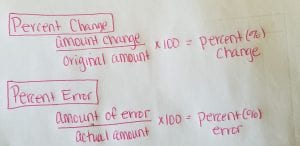# Lesson 13 Vocab

Below are the 4 vocab words for Lesson 13 and the notes that should be on your flip book.

Percent Change- the ratio that compares the amount of change to the original amount.

Percent Increase-  the percent a quantity increases from its original amount.

Percent Decrease- the percent a quantity decreases from its original amount.

Percent Error- the ratio that describes how far an estimate is from the actual amount.